• ## 【知识图谱】——8种Trans模型

万次阅读 多人点赞 2019-03-23 23:56:13
其实 TransA 模型总体来看比较简单，相比较 TransE 模型，本文引入了  Wr  矩阵为不同维度的向量进行加权，并利用 LDL 方法对  Wr  进行分解， Dr  是一个对角阵，对角线上的元素表示不同维度上的权重。本篇...
       知识图谱对实体和它们丰富的关系的结构化信息编码。虽然一个典型的知识图谱可能得到数百万的实体和数以亿计的关系事实，但离补全还很远。知识图谱补全旨在通过现有知识图谱来预测实体之间的关系。知识图谱补全能够找到新的关系事实，这是对从纯文本进行关系抽取的重要补充。知识图谱扫盲：https://www.jiqizhixin.com/articles/2018-06-20-4

在社交网络分析上，知识图谱补全和链接预测很相似，但是由于以下原因知识图谱补有更多的挑战：

（1）知识图谱中的实体具有不同的类型和属性；

（2）知识图谱中的关系具有不同的类型。对于知识图谱补全，我们不仅要判断两个实体之间是否有关系，而且还要预测关系的具体类型。

由于这个原因，传统的链接预测的方法并不能胜任知识图谱补全。近年来，一种很有前景的方法被应用到知识图谱补全，它将知识图谱嵌入到一个连续的向量空间并且保留一定的图中的信息。

下面介绍对知识图谱向量化表示的八种模型做一个介绍：

1、TransE模型

Translating embeddings for modeling multi-relational data

Bordes A, Usunier N, Weston J, et al. NIPS. 2013.

写作动机

之前像 single layer model 以及 NTN 等传统方法，存在着训练复杂和不易拓展等问题。本文提出了一种将实体与关系嵌入到低维向量空间中的简单模型 TransE。该模型已经成为了知识图谱向量化表示的 baseline，并衍生出不同的变体。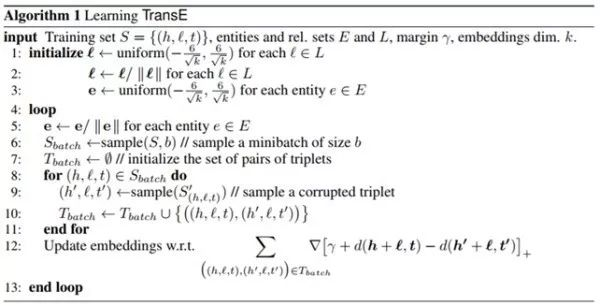正如目标函数所述，算法核心是令正例的 h+r-l 趋近于 0，而负例的 h+r-l 趋近于无穷大，这里的 d 表示 L1 或 L2 范式， \gamma 表示边际距离。整个 TransE 模型的训练过程比较简单，首先对头尾节点以及关系进行初始化，然后每对一个正例取一个负例样本（本文负例选取方式为固定中间的 relation，头尾节点任意替换一个），然后利用 hinge loss function 尽可能使正例和负例分开，最后采用 SGD 方法更新参数。

2、TransH模型

Knowledge Graph Embedding by Translating on Hyperplanes

Wang Z, Zhang J, Feng J, et al. AAAI. 2014.

写作动机

虽然 TransE 模型具有训练速度快、易于实现等优点，但是它不能够解决多对一和一对多关系的问题。以多对一关系为例，固定 r 和 t，TransE 模型为了满足三角闭包关系，训练出来的头节点的向量会很相似。而本文提出一种将头尾节点映射到关系平面的 TransH 模型，能够很好地解决这一问题。

以下图为例，对于多对一关系，TransH 不在严格要求 h+r-l=0，而是只需要保证头结点和尾节点在关系平面上的投影在一条直线上即可，因此能够得到图中头结点向量（红线）正确的表示。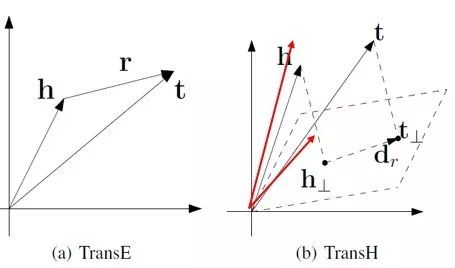模型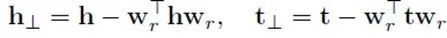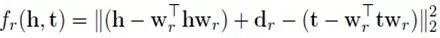本文的模型其实比较简单，首先通过上述公式 1 分别将 head 和 tail 节点映射到关系 r 所对应的平面，之后整个训练方式同 TransE 一致。虽然 TransH 比 TransE 增加了一步向量转换，但其实整体参数只增加了 wr 一项，整体的算法效率还是很高的。

此外，不同于 TransE 模型随机替换 head 和 tail 节点作为负例的方法，本文分别赋给头结点和尾节点一个采样概率，具体计算公式计算如下：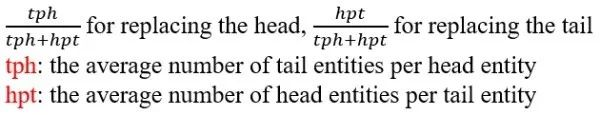即对于多对一的关系，替换尾节点，对于一对多的关系，替换头节点。

3、TransR模型

Learning Entity and Relation Embeddings for Knowledge Graph Completion

Lin Y, Liu Z, Zhu X, et al. AAAI. 2015.

写作动机

TransE 和 TransH 都假设实体和关系嵌入在相同的空间中。然而，一个实体是多种属性的综合体，不同关系对应实体的不同属性，即头尾节点和关系可能不在一个向量空间中。为了解决这个问题，本文提出了一种新的方法 TransR。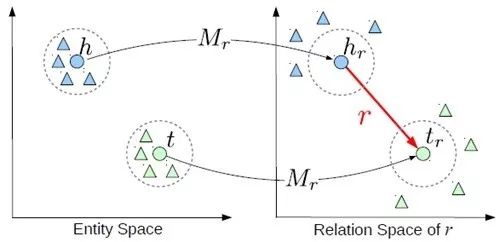模型

TransR 的基本思想如上图所示。对于每个元组（h，r，t），首先将实体空间内的实体通过 Mr 矩阵投影到关系 r 所在的空间内，得到 hr 和 tr ，然后使 hr+t≈tr。特定的关系投影（彩色的圆圈表示）能够使得头/尾实体在这个关系下靠近彼此，使得不具有此关系（彩色的三角形表示）的实体彼此远离。

此外，仅仅通过单个的关系向量还不足以建立从头实体到尾实体的所有转移，即对于同一条关系 r 来讲，r 具有多种语义上的表示。本文提出对不同的头尾实体进行聚类和并且学习到关系在不同聚类簇的表示。作者这里首先利用 TransE 预训练（h，r，t）三元组，并且对 t-h（也就是关系 r）进行聚类，这样对于关系 r 来讲，头尾节点会被分到相应的簇中，并且利用下面的公式进行训练，该方法也被称为 CTransR。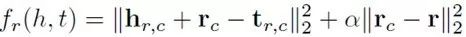4、TransD模型

Learning Entity and Relation Embeddings for Knowledge Graph Completion

Lin Y, Liu Z, Zhu X, et al. AAAI. 2015.

写作动机

之前的 TransE、TransH 和 TransR 都认为每种关系只对应一种语义表示，而在实际情况中，关系r可能代表不同的含义。如下图所示，对于关系 location 来讲，它既可以表示山脉-国家之间的关系，又可以表示为地区-国家之间的关系，因此本文提出一种基于动态矩阵的 TransD 模型来解决这一问题。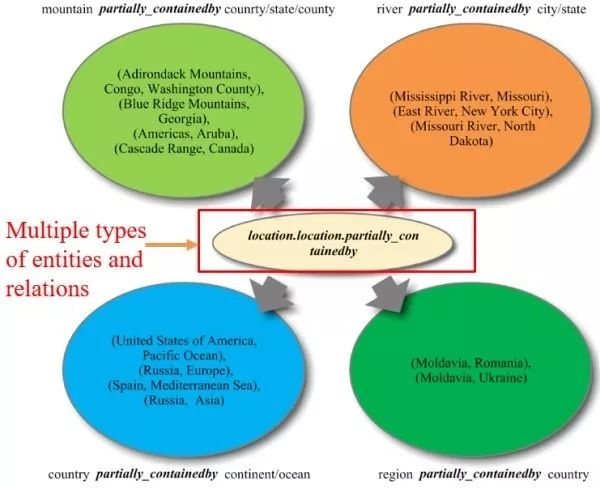模型

TransD 模型同 CTransR 模型一样，都是为了解决关系的多种语义表示。相比较 CTransR 采用聚类的方式，TransD 提出一种动态变化矩阵的方法。具体公式如下图所示：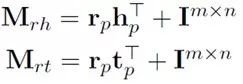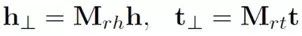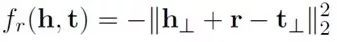对于（h'，r'，t'）两个实体和一种关系来讲，它们分别有两种表示，一种是构建映射矩阵的表示（hp，rp，tp），另外一种是自身的语义表示（h，r，t）。h 和 t 的动态转移矩阵分别由其自身的映射矩阵和关系r的映射矩阵所决定，而不是像 TransR 一样映射矩阵只和关系 r 相关。

此外，该模型的一个亮点在于它将 TransR 的矩阵运算转换成了向量间的运算，运算速度会大幅提升，具体公式如下：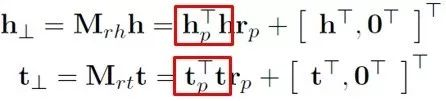5、TransA模型

TransA: An Adaptive Approach for Knowledge Graph Embedding

Xiao H, Huang M, Hao Y, et al. Computer Science. 2015.

写作动机

TransE 从根本上讲是一种欧式距离的计算，其图形对应的是一个圆，从下图可知蓝色部分为正例，红色部分为负例，TransE 模型划分错了七个点。而本文提出了一种基于马氏距离的 TransA 模型，其 PCA 降维图形对应的是一个椭圆，该模型只分错了三个点。

此外，之前的方法将等价对待向量中的每一维，但实际上各个维度的重要性是不同的，只有一些维度是有效的，而某些维度被认为是噪音，会降低效果。

如下图 2 所示，对于关系 haspart 而言，TransE 模型根据欧氏距离计算方法生成了像 Room-has-Goniff 这样的三元组，而正确的结果却是 Room-has-Wall。我们对 x，y 轴进行分解，发现 Room 在 x 轴上距离 Wall 更为相近，因此我们可以认为该图在 x 轴维度上更加重要。TransA 模型通过引入加权矩阵，赋给每一维度权重。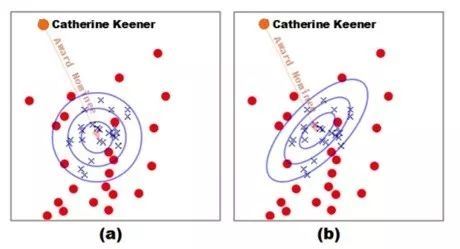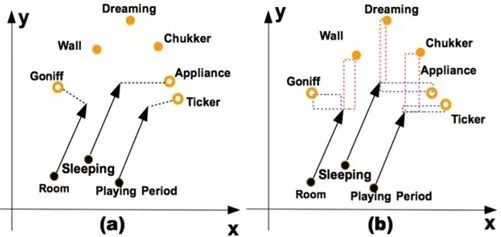模型

其实 TransA 模型总体来看比较简单，相比较 TransE 模型，本文引入了 Wr 矩阵为不同维度的向量进行加权，并利用 LDL 方法对 Wr 进行分解，Dr 是一个对角阵，对角线上的元素表示不同维度上的权重。本篇文章的一个亮点在于通过图像来描述不同的损失度量函数，给人直观的感觉。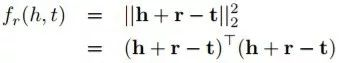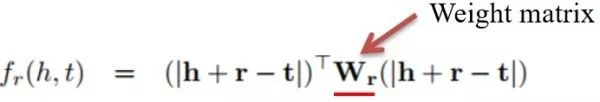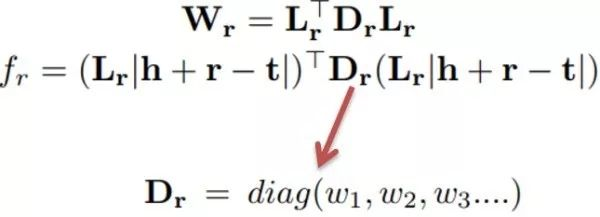6、TransG模型

TransG: A Generative Mixture Model for Knowledge Graph Embedding

Xiao H, Huang M, Zhu X. TransG. ACL. 2016.

写作动机

本文提出的 TransG 模型同 CTransR、TransD 一致，都是为了解决关系的多种语义表达问题。作者首先做了一个比较有意思的实验，首先对预训练好的 t-h 进行聚类（也就是关系 r），发现对于每种关系 r 来讲，会得到不同的簇。从现实意义来看也不难理解，对于关系 Country，我们可以聚类得到美国、欧洲和其他国家这三个簇，表示电影所属的不同国家。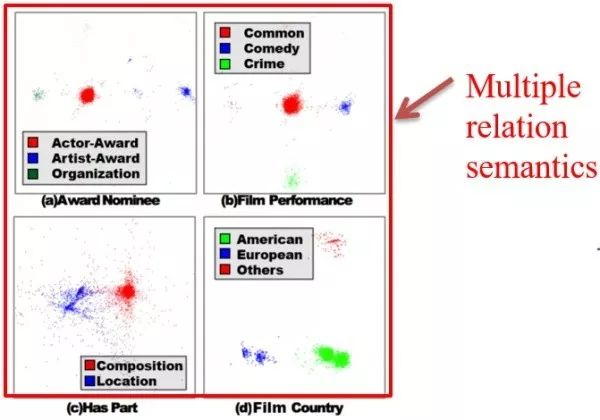模型

TransG 利用贝叶斯非参数混合模型对一个关系生成多个表示，其实 TransG 的本质同 CTransR 一致，都是利用聚类方法生成 r 的不同种表示。不同点在于 CTransR 利用预训练的 TransE 向量进行聚类，而 TransG 采用边训练边聚类的方法。具体公式如下：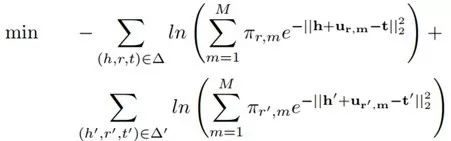M 表示聚类的数目，πr,m 表示关系 r 的第 m 中表示的权重。

7、Transparse模型

Knowledge Graph Completion with Adaptive Sparse Transfer Matrix

Ji G, Liu K, He S, et al. AAAI. 2016.

写作动机

Knowledge Graph 中面临的两个主要问题，分别是 heterogeneous（异构性）和 unbalanced（不平衡性）。

异构性表示知识图谱中关系所连接的节点数目差异较大，不平衡性表示关系所连接的头尾节点数目不同。如果只用一个模型处理所有关系的话可能会导致对简单关系过拟合，对复杂关系欠拟合。本文提出两种模型 Transparse (share) 和 Transparse (separate) 分别解决这两个问题。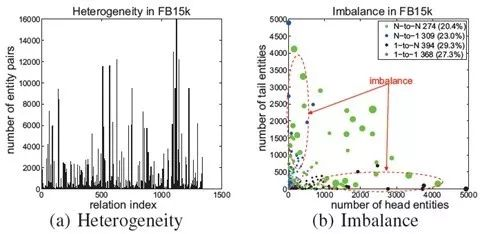模型

Transparse 模型本质是 TransR 模型的扩展，区别在于对于那些复杂的关系令其转移矩阵稠密，而对于简单的关系令其转移矩阵稀疏。这个稀疏程度由 θr 控制，具体计算公式如下：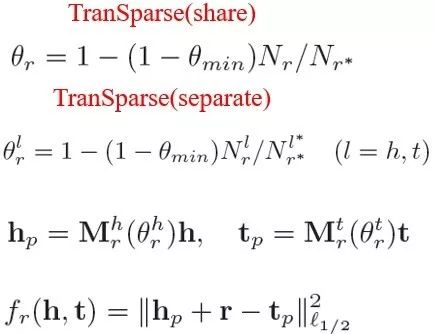θmin 表示 0-1 之间的一个控制变量，Nr 表示关系 r 连接实体对的数量，Nr* 表示其中连接的最大值，同理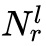表示关系 r 连接的头\尾实体数，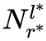表示其中的最大值。

share 和separate 的区别在于 separate 模型为 head 和 tail 提供了两种不同的转移矩阵，而 share 模型 head 和 tail 的转移矩阵相同，具体公式如下所述：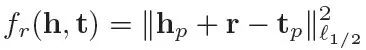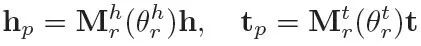该篇文章的亮点在于针对知识图谱存在的异构性和不平衡性问题，作者分别提出两种不同的模型来解决，但缺陷是作者并没有将这两种模型进行融合，最后在 triplet classification 和 link prediction 上的结果并没有比 TransD 提升很多。

8、TransA+模型

Locally Adaptive Translation for Knowledge Graph Embedding

Jia Y, Wang Y, Lin H, et al. AAAI, 2016.

写作动机

之前所有模型的 margin 都是固定不变的，但局部不同的 margin 是否能够提升模型的效果呢？作者为了证明，首先做了一组实验，将 FB15K 分成两个子集，在不同数据集上分别得到达到最优效果的 margin，发现局部最优的 margin 不尽相同。

从理论来讲，Margin 变大，更多的数据被训练，容易导致过拟合。相反，margin 越小，容易欠拟合。所以也证明了 margin 对最终结果的影响。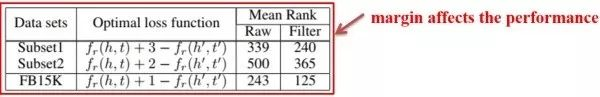模型

本文将 margin 分为 entity 和 relation 两部分，并利用线性插值进行组合。实体部分的 margin 应保证内层圆包含更多的正例，外层圆的外部应尽可能是负例。对于 relation 部分，本文利用 relation 的长度，即 L2 范式来衡量相似度关系。具体公式如下图所示：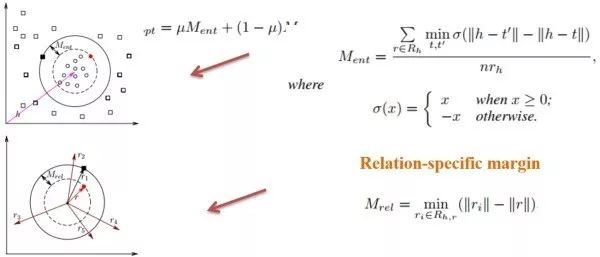Rh,r 是除了关系 r 之外的所有关系的集合，它使得所有与关系 r 不相近的关系远离 r。本篇文章比较巧妙地利用局部 margin 的方式，在 link prediction 和 triple classification 结果上有了明显提升。

参考文献：

Bordes A, Usunier N, Weston J, et al. Translating embeddings for modeling multi-relational data. NIPS. 2013.
Wang Z, Zhang J, Feng J, et al. Knowledge Graph Embedding by Translating on Hyperplanes. AAAI. 2014.
Lin Y, Liu Z, Zhu X, et al. Learning Entity and Relation Embeddings for Knowledge Graph Completion. AAAI. 2015.
Lin Y, Liu Z, Zhu X, et al.Learning Entity and Relation Embeddings for Knowledge Graph Completion. AAAI. 2015.
Xiao H, Huang M, Hao Y, et al. TransA: An Adaptive Approach for Knowledge Graph Embedding. Computer Science. 2015.
Xiao H, Huang M, Zhu X. TransG: A Generative Mixture Model for Knowledge Graph Embedding. ACL. 2016.
Ji G, Liu K, He S, et al. Knowledge Graph Completion with Adaptive Sparse Transfer Matrix. AAAI. 2016.
Jia Y, Wang Y, Lin H, et al. Locally Adaptive Translation for Knowledge Graph Embedding. AAAI, 2016.


展开全文• 文章转载：【知识图谱】——8种Trans模型 基于翻译模型(Trans系列)的知识表示学习 TransE解释
文章转载：【知识图谱】——8种Trans模型

基于翻译模型(Trans系列)的知识表示学习

TransE解释


展开全文知识图谱
• TransE模型的基本思想是使head向量和relation向量的和尽可能靠近tail向量。这里我们用L1或L2范数来衡量它们的靠近程度。 损失函数是使用了负抽样的max-margin函数。 L(y, y’) = max(0, margin - y + y’) y是正...
模型介绍
TransE模型的基本思想是使head向量和relation向量的和尽可能靠近tail向量。这里我们用L1或L2范数来衡量它们的靠近程度。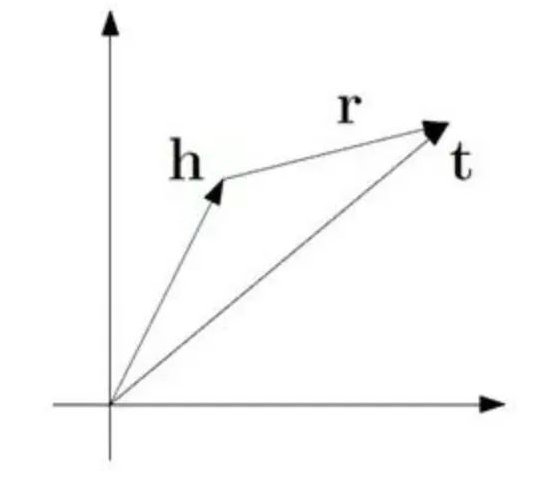损失函数是使用了负抽样的max-margin函数。
L(y, y’) = max(0, margin - y + y’)
y是正样本的得分，y'是负样本的得分。然后使损失函数值最小化，当这两个分数之间的差距大于margin的时候就可以了(我们会设置这个值，通常是1)。
由于我们使用距离来表示得分，所以我们在公式中加上一个减号，知识表示的损失函数为：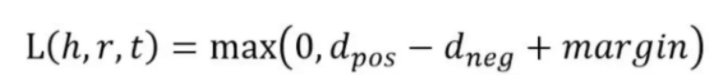其中，d是：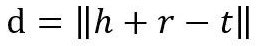这是L1或L2范数。至于如何得到负样本，则是将head实体或tail实体替换为三元组中的随机实体。

代码实现：
具体的代码和数据集（YAGO、umls、FB15K、WN18）请见Github：
https://github.com/Colinasda/TransE.git
import codecs
import numpy as np
import copy
import time
import random

entities2id = {}
relations2id = {}

def dataloader(file1, file2, file3):
print("load file...")

entity = []
relation = []
with open(file2, 'r') as f1, open(file3, 'r') as f2:
lines1 = f1.readlines()
lines2 = f2.readlines()
for line in lines1:
line = line.strip().split('\t')
if len(line) != 2:
continue
entities2id[line] = line
entity.append(line)

for line in lines2:
line = line.strip().split('\t')
if len(line) != 2:
continue
relations2id[line] = line
relation.append(line)

triple_list = []

with codecs.open(file1, 'r') as f:
content = f.readlines()
for line in content:
triple = line.strip().split("\t")
if len(triple) != 3:
continue

h_ = entities2id[triple]
r_ = relations2id[triple]
t_ = entities2id[triple]

triple_list.append([h_, r_, t_])

print("Complete load. entity : %d , relation : %d , triple : %d" % (
len(entity), len(relation), len(triple_list)))

return entity, relation, triple_list

def norm_l1(h, r, t):
return np.sum(np.fabs(h + r - t))

def norm_l2(h, r, t):
return np.sum(np.square(h + r - t))

class TransE:
def __init__(self, entity, relation, triple_list, embedding_dim=50, lr=0.01, margin=1.0, norm=1):
self.entities = entity
self.relations = relation
self.triples = triple_list
self.dimension = embedding_dim
self.learning_rate = lr
self.margin = margin
self.norm = norm
self.loss = 0.0

def data_initialise(self):
entityVectorList = {}
relationVectorList = {}
for entity in self.entities:
entity_vector = np.random.uniform(-6.0 / np.sqrt(self.dimension), 6.0 / np.sqrt(self.dimension),
self.dimension)
entityVectorList[entity] = entity_vector

for relation in self.relations:
relation_vector = np.random.uniform(-6.0 / np.sqrt(self.dimension), 6.0 / np.sqrt(self.dimension),
self.dimension)
relation_vector = self.normalization(relation_vector)
relationVectorList[relation] = relation_vector

self.entities = entityVectorList
self.relations = relationVectorList

def normalization(self, vector):
return vector / np.linalg.norm(vector)

def training_run(self, epochs=1, nbatches=100, out_file_title = ''):

batch_size = int(len(self.triples) / nbatches)
print("batch size: ", batch_size)
for epoch in range(epochs):
start = time.time()
self.loss = 0.0
# Normalise the embedding of the entities to 1
for entity in self.entities.keys():
self.entities[entity] = self.normalization(self.entities[entity]);

for batch in range(nbatches):
batch_samples = random.sample(self.triples, batch_size)

Tbatch = []
for sample in batch_samples:
corrupted_sample = copy.deepcopy(sample)
pr = np.random.random(1)
if pr > 0.5:
# change the head entity
corrupted_sample = random.sample(self.entities.keys(), 1)
while corrupted_sample == sample:
corrupted_sample = random.sample(self.entities.keys(), 1)
else:
# change the tail entity
corrupted_sample = random.sample(self.entities.keys(), 1)
while corrupted_sample == sample:
corrupted_sample = random.sample(self.entities.keys(), 1)

if (sample, corrupted_sample) not in Tbatch:
Tbatch.append((sample, corrupted_sample))

self.update_triple_embedding(Tbatch)
end = time.time()
print("epoch: ", epoch, "cost time: %s" % (round((end - start), 3)))
print("running loss: ", self.loss)

with codecs.open(out_file_title +"TransE_entity_" + str(self.dimension) + "dim_batch" + str(batch_size), "w") as f1:

for e in self.entities.keys():
# f1.write("\t")
# f1.write(e + "\t")
f1.write(str(list(self.entities[e])))
f1.write("\n")

with codecs.open(out_file_title +"TransE_relation_" + str(self.dimension) + "dim_batch" + str(batch_size), "w") as f2:
for r in self.relations.keys():
# f2.write("\t")
# f2.write(r + "\t")
f2.write(str(list(self.relations[r])))
f2.write("\n")

def update_triple_embedding(self, Tbatch):
# deepcopy 可以保证，即使list嵌套list也能让各层的地址不同， 即这里copy_entity 和
# entitles中所有的elements都不同
copy_entity = copy.deepcopy(self.entities)
copy_relation = copy.deepcopy(self.relations)

for correct_sample, corrupted_sample in Tbatch:

correct_copy_head = copy_entity[correct_sample]
correct_copy_tail = copy_entity[correct_sample]
relation_copy = copy_relation[correct_sample]

corrupted_copy_head = copy_entity[corrupted_sample]
corrupted_copy_tail = copy_entity[corrupted_sample]

correct_head = self.entities[correct_sample]
correct_tail = self.entities[correct_sample]
relation = self.relations[correct_sample]

corrupted_head = self.entities[corrupted_sample]
corrupted_tail = self.entities[corrupted_sample]

# calculate the distance of the triples
if self.norm == 1:
correct_distance = norm_l1(correct_head, relation, correct_tail)
corrupted_distance = norm_l1(corrupted_head, relation, corrupted_tail)

else:
correct_distance = norm_l2(correct_head, relation, correct_tail)
corrupted_distance = norm_l2(corrupted_head, relation, corrupted_tail)

loss = self.margin + correct_distance - corrupted_distance

if loss > 0:
self.loss += loss
print(loss)
correct_gradient = 2 * (correct_head + relation - correct_tail)
corrupted_gradient = 2 * (corrupted_head + relation - corrupted_tail)

if self.norm == 1:
for i in range(len(correct_gradient)):
if correct_gradient[i] > 0:
correct_gradient[i] = 1
else:
correct_gradient[i] = -1

if corrupted_gradient[i] > 0:
corrupted_gradient[i] = 1
else:
corrupted_gradient[i] = -1

correct_copy_head -= self.learning_rate * correct_gradient
relation_copy -= self.learning_rate * correct_gradient
correct_copy_tail -= -1 * self.learning_rate * correct_gradient

relation_copy -= -1 * self.learning_rate * corrupted_gradient
if correct_sample == corrupted_sample:
# if corrupted_triples replaces the tail entity, the head entity's embedding need to be updated twice
correct_copy_head -= -1 * self.learning_rate * corrupted_gradient
corrupted_copy_tail -= self.learning_rate * corrupted_gradient
elif correct_sample == corrupted_sample:
# if corrupted_triples replaces the head entity, the tail entity's embedding need to be updated twice
corrupted_copy_head -= -1 * self.learning_rate * corrupted_gradient
correct_copy_tail -= self.learning_rate * corrupted_gradient

# normalising these new embedding vector, instead of normalising all the embedding together
copy_entity[correct_sample] = self.normalization(correct_copy_head)
copy_entity[correct_sample] = self.normalization(correct_copy_tail)
if correct_sample == corrupted_sample:
# if corrupted_triples replace the tail entity, update the tail entity's embedding
copy_entity[corrupted_sample] = self.normalization(corrupted_copy_tail)
elif correct_sample == corrupted_sample:
# if corrupted_triples replace the head entity, update the head entity's embedding
copy_entity[corrupted_sample] = self.normalization(corrupted_copy_head)
# the paper mention that the relation's embedding don't need to be normalised
copy_relation[correct_sample] = relation_copy
# copy_relation[correct_sample] = self.normalization(relation_copy)

self.entities = copy_entity
self.relations = copy_relation

if __name__ == '__main__':
file1 = "/umls/train.txt"
file2 = "/umls/entity2id.txt"
file3 = "/umls/relation2id.txt"

entity_set, relation_set, triple_list = dataloader(file1, file2, file3)

# modify by yourself
transE = TransE(entity_set, relation_set, triple_list, embedding_dim=30, lr=0.01, margin=1.0, norm=2)
transE.data_initialise()
transE.training_run(out_file_title="umls_")




展开全文python 知识图谱
• 知识图谱——TransE模型原理 1 TransE模型介绍 1.1 TransE模型引入 在我们之前的文章中，提到了知识图谱补全任务的前提任务是知识表示学习，在知识表示学习中，最为经典的模型就是TransE模型TransE模型的核心作用...
知识图谱——TransE模型原理
1 TransE模型介绍
1.1 TransE模型引入
在我们之前的文章中，提到了知识图谱补全任务的前提任务是知识表示学习，在知识表示学习中，最为经典的模型就是TransE模型，TransE模型的核心作用就是将知识图谱中的三元组翻译成embedding向量。
1.2 TransE模型思想
为了后面便于表示，我们先设定一些符号

h 表示知识图谱中的头实体的向量。
t 表示知识图谱中的尾实体的向量。
r 表示知识图谱中的关系的向量。

在TransE模型中，有这样一个假设：
$t=h+r$
也就是说，正常情况下的尾实体向量=头实体向量+关系向量。用图的方式描述如下：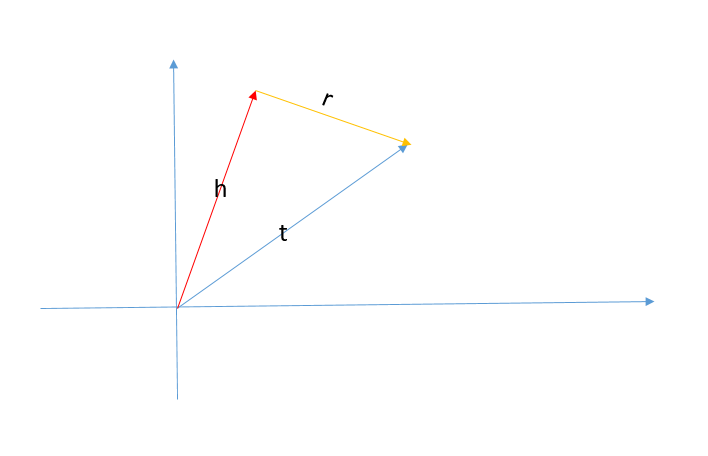如果一个三元组不满足上述的的关系，我们就可以认为这是一个错误的三元组。
1.3 TransE模型的目标函数
首先，我们先来介绍两个数学概念：
L1范数
也称为曼哈顿距离，对于一个向量X而言，其L1范数的计算公式为：
$||X||_{L1}=∑_{i=1}^n|x_i|$
其中，$x_i$表示向量X的第i个属性值，这里我们取的是绝对值。并且，使用L1范数可以衡量两个向量之间的差异性，也就是两个向量的距离。
$Distance_{L1}(X_1,X_2)=∑_{i=1}^n|X_{1i}-X_{2i}|$
L2范数
也称为欧式距离，对于一个向量X而言，其L2范数的计算公式为：
$||X||_{L2}=\sqrt{∑_{i=1}^nX_i^2}$
同样，L2范数也可以用来衡量两个向量之间的差距:
$Distance_{L2}(X_1,X_2)=∑_{i=1}^n(X_{1i}-X_{2i})^2$
根据我们上面介绍的Trans中的假设，我们可以知道，对于一个三元组而言，头实体向量和关系向量之和与尾实体向量越接近，那么说明该三元组越接近一个正确的三元组，差距越大，那么说明这个三元组越不正常。那么我们可以选择L1或者L2范数来衡量三个向量的差距。而我们的目标就是使得争取的三元组的距离越小越好，错误的三元组距离越大越好，也就是其相反数越小越好。数学化的表示就是：
$min∑_{(h,r,t)∈G}∑_{(h',r',t')∈G'}[γ+distance(h+r,t)-distance(h'+r',t')]_+$
其中：
$(h,r,t)$：表示正确的三元组
$(h',r',t')$： 表示错误的三三元组
$γ$ ：表示正样本和负样本之间的间距，一个常数
$[x]_+$：表示max(0,x)
我们来简单的解释以下目标函数，我们的目标是让正例的距离最小，也就是$min(distance(h+r,t))$，让负例的相反数最小也就是$(min(-distance(h'+r',t')))$，对于每一个正样本和负样本求和，再增加一个常数的间距，就是整体距离的最小值。也就是我们的目标函数。
1.4 目标函数的数学推导
这里，我们采用欧氏距离作为distance函数，则目标函数可以改写为：
$min∑_{(h,r,t)∈G}∑_{(h',r',t')∈G'}[γ+(h+r-t)^2-(h'+r'-t')^2]_+$
则对于损失函数loss就有：
$Loss = ∑_{(h,r,t)∈G}∑_{(h',r',t')∈G'}[γ+(h+r-t)^2-(h'+r-t')^2]_+$
在损失函数中，我们知道所有的参数包括{$h,r,t,h',r,t'$}。下面，我们来逐个进行梯度推导：

首先是对h的梯度，对于某一个$h_i$而言
$\frac{∂Loss}{∂h_i}= ∑_{(h_,r,t)∈G}∑_{(h',r,t')∈G'}\frac{∂[γ+(h+r-t)^2-(h'+r-t')^2]_+}{∂h_i}$
在整个求和的过程中，只针对包含$h_i$的项求导：
$\frac{∂[γ+(h_i+r-t)^2-(h'+r-t')^2]_+}{∂h_i}$
有：
$\frac{∂[γ+(h_i+r-t)^2-(h'+r-t')^2]_+}{∂h_i}= \begin{cases} 2(h_i+r-t)&&γ+(h+r-t)^2-(h'+r-t')^2>0\\ 0 &&γ+(h+r-t)^2-(h'+r-t')^2<=0 \end{cases}$
则原式变为：
$\frac{∂Loss}{∂h_i}= ∑_{(h_i,r,t)∈G}∑_{(h',r,t')∈G'} \begin{cases} 2(h_i+r-t)&&γ+(h_i+r-t)^2-(h'+r-t')^2>0\\ 0 &&γ+(h_i+r-t)^2-(h'+r-t')^2<=0 \end{cases}$
同理对于$t_i,h_i',t_i'$有：
$\frac{∂Loss}{∂t_i}= ∑_{(h,r,t_i)∈G}∑_{(h',r,t')∈G'} \begin{cases} -2(h+r-t_i)&&γ+(h+r-t_i)^2-(h'+r-t')^2>0\\ 0 &&γ+(h+r-t_i)^2-(h'+r-t')^2<=0 \end{cases}$
$\frac{∂Loss}{∂h_i'}= ∑_{(h,r,t)∈G}∑_{(h_i',r,t')∈G'} \begin{cases} -2(h'+r-t')&&γ+(h+r-t)^2-(h_i'+r-t')^2>0\\ 0 &&γ+(h+r-t)^2-(h_i'+r-t')^2<=0 \end{cases}$
$\frac{∂Loss}{∂t_i'}= ∑_{(h,r,t)∈G}∑_{(h',r,t_i')∈G'} \begin{cases} 2(h'+r-t')&&γ+(h+r-t)^2-(h'+r-t_i')^2>0\\ 0 &&γ+(h+r-t)^2-(h'+r-t_i')^2<=0 \end{cases}$
最后对于$r_i$，γ有：
$\frac{∂Loss}{∂r_i}=∑_{(h,r_i,t)∈G}∑_{(h',r_i,t')∈G'} \begin{cases} 2(h+r_i-t)-2(h'+r_i-t')&&γ+(h+r_i-t)^2-(h'+r_i-t')^2>0\\ 0 &&γ+(h+r_i-t)^2-(h'+r_i-t')^2<=0 \end{cases}$
$\frac{∂Loss}{∂γ}= ∑_{(h,r,t)∈G}∑_{(h',r',t')∈G'} \begin{cases} 1&&γ+(h+r-t)^2-(h'+r-t')^2>0\\ 0 &&γ+(h+r-t)^2-(h'+r-t')^2<=0 \end{cases}$

1.5 如何产生负样本
在我们之前算法描述中，我们提到了负样本的问题，对于一个知识图谱而言，其中保存的全部都是正样本时肯定的了。那么，我们应该如何获取负样本呢？
具体的可以通过随机替换头实体的方式来实现一个错误的三元组，或者采用随机替换一个错误的尾实体的方式来形成一个错误的三元组。
同时，为了避免，我们在替换形成的三元组也存在于知识图谱中，我们需要在替换之后进行过滤。
2 参考文章

TransE如何进行向量更新？
TransE算法详解


展开全文• TransE模型-数据预处理源代码数据说明代码解释 源代码 源代码参考项目 数据说明 数据集采用FB15K，下面代码中的文件分别为： file1：训练集，格式为（head，relation，tail） 例： /m/027rn /location/country/form_...python 知识图谱
• trans系列模型看了好久，但苦恼了好几天，不知道怎么用自己的数据训练模型。今天仔细看fb15k和几个其他的公开数据，终于完成了！多琢磨几个公共数据的共性就可以了.一定要多看几个公共数据集！ ...
• TransE、TransH模型都是图谱中关于实体嵌入的模型，它们都是基于向量平移的模型。 它们的目的是使 h+r=t，所以它们的损失函数是比较h+r和t之间的距离,目的是使所有三元组的h+r与t的距离之和最小,我们可以定义h+r与t...算法 自然语言处理
• TransE训练后模型的理想状态是，从实体矩阵和关系矩阵中各自抽取一个向量，进行L1或者L2运算，得到的结果近似于实体矩阵中的另一个实体的向量，从而达到通过词向量表示知识图谱中已存在的三元组 的关系。 TransE...
• TransE模型属于翻译模型：直观上，将每个三元组实例（head，relation，tail）中的关系relation看做从实体head到实体tail的翻译，通过不断调整h、r 和 t（head、relation和tail的向量），使（h + r） 尽可能与 t 相等...知识图谱 表示学习
• 翻译模型Trans） 解决问题：知识表示与推理 将实体向量表示（Embedding）在低维稠密向量空间中，然后进行计算和推理。 主要应用：triplet classification, link prediction 目前基于翻译模型Trans系列...知识表示学习 知识图谱 关系三元组
• 1.canonical model（典范模型） canonical这个词来源于canon。 没错，就是和洗脑神曲卡农同源。canon本身也是拉丁语单词，意思是律法，带有一定的宗教意味。所以canonical，形象地来说可以解释为是 天选的。 如果一...
• 本文介绍了TransE，TransH，TransR，TransD，RotatE知识表示嵌入模型的基本概念和评价函数。 TransE 论文标题：Translating Embeddings for Modeling Multi-relational Data (2013) TransE将relation看作head到tail...知识图谱
• TransE模型 TransE认为在知识库中，三元组<h,r,t>可以看成头实体h到尾实体t利用关系r所进行的翻译。 比如，<柏拉图，老师，苏格拉底>头实体“柏拉图”的向量加上关系“老师”的向量，应该尽可能和尾...
• 给定一个由三元组(h, l, t)组成的训练集S，其中h，t属于E，关系属于L，我们的模型学习实体和关系的向量嵌入。嵌入的取值属于（k是模型的一个参数），用相同的大写字母表示。
• TransE之前，有Structed Embedding, Semantic Matching Energy等模型，在TransE之后，人们在此基础上进行改进，依次提出了TransH, TransR, TransD等模型。接线来介绍基于TransE的改进模型。 作者：孙天祥 链接...
• 作者：Xu LIANG编译：ronghuaiyang来自：AI公园导读一文打尽图嵌入Translate模型，各种模型的动机，优缺点分析。本文对知识图谱嵌入/知识表示的转换模型进行了简要的...
• ## TransE

2019-07-17 20:15:59
一、TransE提出了一种将实体与关系嵌入到低维向量空间中的简单模型 二、具体算法
• 接下来，我们将对Satoshi Iizuka等人的这篇文献[ACM Trans. Graph. 35, 4, Article 110 (July 2016)]关于灰度图上色进行分析。 论文亮点 该论文的亮点包括： 可以有效混合灰度图中物体的局域特征与全局特征...
• ## TransE算法

千次阅读 2018-04-11 18:35:59
TransE算法中存在一个设定，它将关系看作是实体间的平移向量，也就是说对于一个三元组（h,r,t）对应的向量lh，lr，lt，希望 lh+lr =lt 这源于Mikolov等人在2013年提出的word2vec词表示学习模型，他们发现词向量...
• TransE的直观含义，就是TransE基于实体和关系的分布式向量表示，将每个三元组实例（head，relation，tail）中的关系relation看做从实体head到实体tail的翻译（其实我一直很纳闷为什么叫做translating，其实就是向量...知识图谱推理
• 七参数转换(布尔沙模型) 大地坐标空间坐标互转 高斯正反算(在中国一般把X轴向东移动500km,本程序将带号也加在了Y坐标前面) 一些常见的曲率半径计算 支持的参考椭球: WGS84 CGCS2000 西安80(国际1975椭球) coordinate...C#
• ## Trans系列文章解读

千次阅读 2018-03-25 16:31:09
本系列，笔者转载搜狐（机器之心博主）的文章，如有侵权，联系笔者立即删除-----------------------------分割线-------------------------------------本期PaperWeekly的主题是基于翻译模型(Trans系列)的知识表示...知识图谱
• ## 【论文解读】TransE

千次阅读 2019-07-05 23:45:27
Trans系列的主题是基于翻译模型的知识表示学习，主要用来解决知识表示和推理的问题。本文主要介绍TransE和数据集Wordnet、Freebase等。 表示学习：主要面向知识图谱中实体和关系进行表示学习，一般使用建模方法将...机器学习 知识图谱 NLP...